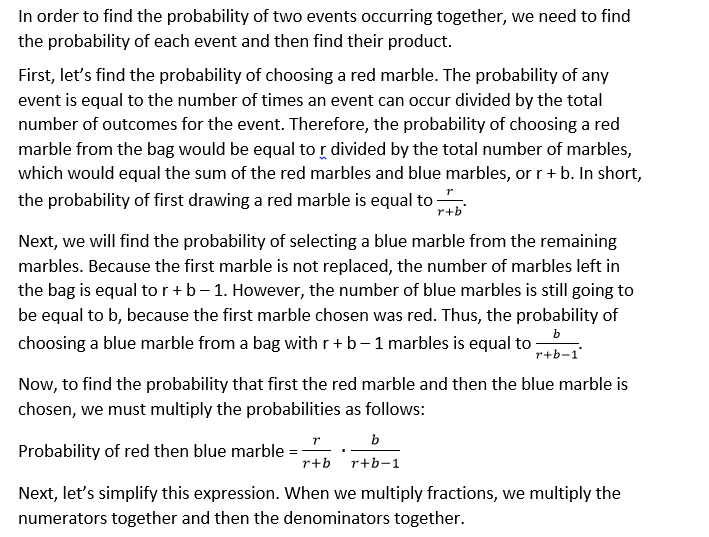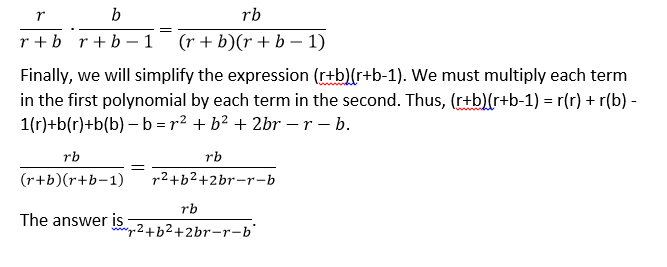## Example Questions

### Example Question #31 : Probability

Let R = {1, 3, 4, 12}, and let Q = {2, 6, 8, 24}. If one number is randomly selected from R, and another is randomly selected from Q, what is the probability that the product of those two randomly chosen numbers will belong to Q?

1/2

1/4

9/16

5/8

5/16

9/16

Explanation:

First, let's consider all of the pairs that could be chosen from R and Q. There are sixteen possibilities:

1 and 2; 1 and 6; 1 and 8; 1 and 24; 3 and 2; 3 and 6; 3 and 8; 3 and 24; 4 and 2; 4 and 6; 4 and 8; 4 and 24; 12 and 2; 12 and 6; 12 and 8; 12 and 24.

Now, we need to find the product of each of these pairs, and then determine whether or not they belong to Q. The products of all of the possible pairs would be as follows:

1(2) = 2, which belongs to Q

1(6) = 6, which belongs to Q

1(8) = 8, which belongs to Q

1(24) = 24, which belongs to Q

3(2) = 6, which belongs to Q

3(6) = 18, which doesn't belong to Q

3(8) = 24, which belongs to Q

3(24) = 72, which doesn't belong

4(2) = 8, which belongs to Q

4(6) = 24, which belongs to Q

4(8) = 32, which doesn't belong

4(24) = 96, which doesn't belong

12(2) = 24, which belongs to Q

12(6) = 72, which doesn't belong

12(8) = 96, which doesn't belong

12(24) = 288, which doesn't belong

Thus, of our sixteen possible combinations, there are 9 which, when multiplied, give a number that belongs to Q. Because probability is the number of successful events out of the total number of events, the probability is 9/16.

### Example Question #32 : Probability

1) If John does not have freckles, he is not Tim's sibling.

2) If Jake does not have freckles, he is Tim's sibling.

3) If Suzy is not Tim's sibling, she will not have freckles.

4) All of the other answers are false.

All of Tim's siblings have freckles. Which of the above statements must be true?

2) If Jake does not have freckles, he is Tim's sibling.

3) If Suzy is not Tim's sibling, she will not have freckles.

4) All of the other answers are false.

1) If John does not have freckles, he is not Tim's sibling.

1) If John does not have freckles, he is not Tim's sibling.

Explanation:

Only John's statement must be true. Suzy could have freckles and not be Tim's sibling and if Jake doesn't have freckles then he is not Tim's sibling.

### Example Question #131 : Statistics

A bag contains red marbles and blue marbles only. Let r equal the number of red marbles and b equal the number of blue marbles in the bag. Mark draws one marble from the bag and then a second one without replacing the first. Which of the following expressions is equivalent to the probability that Mark will draw a red marble and then a blue marble?

(r + b – 1)/(r+ 2br + b2)

(rb – r – b)/(r+ b+ 2br – r – b)

rb/(r+ b2)

rb/(r+ 2br + b2)

rb/(r+ b+ 2br – r – b)

rb/(r+ b+ 2br – r – b)

Explanation:### Example Question #132 : Statistics

In a bag, there are 5 blue marbles, 3 red marbles, and 2 green marbles.  If three marbles are chosen consecutively at random without replacement, what is the probability that the color of the chosen marbles would be blue-red-green in that order?

1/24

1/300

3/100

1/3

1/1000

1/24

Explanation:

The probability that a blue marble is chosen first is 5/10 because at the outset there are 5 blue marbles in a total of 10 marbles.  After one marble has been chosen, there are 9 total marbles remaining since there is no replacement.  The probability that a red marble is chosen in the second pick is 3/9 (because there are 3 red marbles out of a total of 9).  There are now 8 total marbles remaining and 2 green marbles. Thus, the probability of picking a green marble on the third pick is 2/8.

Therefore, the probability of picking a blue-red-green marble outcome (in that order) is:

5/10 * 3/9 * 2/8 = 30/720 = 1/24

### Example Question #133 : Statistics

A book shelf has 15 theology books, 20 philosophy books, and 5 history books. If someone were to draw two texts at random, what is the chance that they would draw at least one theology book?

5/13

44/121

9/64

8/13

8/13

Explanation:

Thinking through our data, we know that we are looking for the following combination of events, where T is a theology book, and < > represents a draw from the shelf:

1. <T><T>

2. <T><Non-T>

3. <Non-T><T>

To save us quite a bit of trouble, let us note that only one event is excluded:

<Non-T><Non-T

The easiest way to solve this would be to solve for the probability of this one case and subtract that from 1. This will give us the "remaining probability" that applies to the three cases that we want.

The <Non-T><Non-T> case would be calculated:

First draw: 25/40 = 5/8

Second draw: 24/39 = 8/13

Total probability: (5/8) * (8/13) = 5/13

The probability of our case is 1 – (5/13) = (13 – 5)/13 = 8/13.

### Example Question #134 : Statistics

A drawing is being held for concert tickets at a high school. There are 200 seniors, 150 juniors, 200 sophomores and 150 freshmen. Each senior's name is placed in the drawing 4 times, each junior's 3, sophomores 2, and freshmen one time. What is the probability that a senior's name will be chosen?

2/7

2/9

2/9

4/9

5/9

4/9

Explanation:

You multiply each number of students in each class by how many times that class is entered into the drawing, then add up the totals. There will be a total of 1800 entrants and 800 will be seniors.

### Example Question #135 : Statistics

Set S = {0, 1, 5, 9}, and set T = {2, 3, 4, 7}. If one number is chosen randomly from S and another is chosen randomly from T, what is the probability that the sum of these two numbers will be prime?

5/8

1/2

9/16

1/4

7/16

1/2

Explanation:

There are four possible numbers that can be chosen from S, and there are four that can be chosen from T. This means that there are 4 * 4, or 16, pairs of numbers that can be drawn from S and T. We need to find the sum of these pairs and determine if each sum is prime. Let's find the sum of the 16 pairs. Remember that a number is prime if it divisible only by itself and 1.

0 + 2 = 2, which is prime

0 + 3 = 3, prime

0 + 4 = 4, not prime

0 + 7 = 7, prime

1 + 2 = 3, prime

1 + 3 = 4, not

1 + 4 = 5, prime

1 + 7 = 8, not

5 + 2 = 7, prime

5 + 3 = 8, not

5 + 4 = 9, not

5 + 7 = 12, not

9 + 2=11, prime

9 + 3 = 12, not

9 + 4 = 13, prime

9 + 7 = 16, not

Of the sixteen pairs, 8 have a sum that equals a prime number. Thus, because each of these pairs has an equal chance of being drawn randomly, the probability that the sum will be prime is 8 out of 16, or 8/16 = 1/2.

### Example Question #136 : Statistics

Judy is practicing to be a magician and has an ordinary deck of 52 playing cards, a regular 6-sided die, and a fair coin. What is the probability that Judy rolls a 6 on the die, then flips the coin head face up, and then draws a spade from the deck of cards?

3/16

1/48

2/25

1/4

11/12

1/48

Explanation:

We can find the individual probabilities of these three events occuring first.

P(rolling a 6) = 1/6

Now, to find the probability of rolling a 6 AND flipping a head AND drawing a spade, we must multiply the individual probabilities. So the answer is 1/6 * 1/2 * 1/4 = 1/48.

### Example Question #137 : Statistics

A bag of marbles has 7 yellow marbles, 5 red marbles, 3 blue marbles, and 6 white marbles. What is the probability of choosing a yellow marble, putting it back and choosing a blue marble, and then NOT putting the blue marble back and picking a white marble?

3/17

8/31

2/3

1/9

1/70

1/70

Explanation:

There are a total of 7 + 5 + 3 + 6 = 21 marbles. The probability of picking a yellow marble is 7/21 = 1/3. Then we put it back and choose a blue marble with probability 3/21 = 1/7. We do NOT put this blue marble back, but then we grab for a white. The probability of picking a white is now 6/20 = 3/10, because now we are choosing from 20 marbles instead of 21. So putting it together, the probability of choosing a yellow marble, replacing it and then choosing a blue and a white, is 1/3 * 1/7 * 3/10 = 1/70.

### Example Question #138 : Statistics

In a regular 52-card deck, what is the probability of drawing three aces in a row, with replacement?

1/52 * 1/52 * 1/52

1/52 + 1/52 + 1/52

4/52 * 4/52 * 4/52

1/52 * 1/51 * 1/50

13/52 * 13/51 * 13/50